Latest Banking jobs   »

# Reasoning Ability Quiz For IBPS Clerk Prelims 2022- 12th July

Q1. If it is possible to make a meaningful word from 1st, 2nd, 4th, 5th and 6th letters of word “EMPIRICAL“, then which will be the 2nd letter of the word from left end? If no such word can be formed, then mark the answer as ‘Y’. If more than one word can be formed mark the answer as ‘X’.
(a) Y
(b) M
(c) E
(d) I
(e) X

Reasoning Quiz For IBPS Clerk Prelims 2022 12th July CHECK IN HINDI

Q2. How many pairs of letters are there in the word “TURBULENCE” which has as many letters between them in the word as in the alphabetical series (Both forward and backward direction)?
(a) One
(b) Two
(c) Three
(d) Four
(e) More than four

Q3. If all the even digits of the number “564854538” are decreased by ‘1’ and then all the digits are arranged in ascending order from left to right, then what will be the sum of fifth digit from left end and third digit from the right end?
(a) 3
(b) 4
(c) 6
(d) 8
(e) None of these

Q4. If all the consonants of the Word “ACTUATE” are replaced by immediately preceding letter & vowels to its immediately following letter in the alphabetical series, how many vowels are there in the rearranged word?
(a) Two
(b) Three
(c) Four
(d) Five
(e) None

Q5. How many pairs of letters are there in the word “ENDURANCE” which has as many letters between them in the word as in the alphabetical series (Both forward and backward direction)?
(a) One
(b) Two
(c) Three
(d) Four
(e) More than four

Q6. How many pairs of letters are there in the word “CONSTANCY” which has as many letters between them in the word as in the alphabetical series (Both forward and backward direction)?
(a) One
(b) Two
(c) Three
(d) Four
(e) More than four

Q7. If all the odd digits of the number “746587835” are increased by ‘1’ and then all the digits are arranged in descending order from left to right, then what will be the difference of fifth digit from left end and third digit from the right end?
(a) 3
(b) 4
(c) 0
(d) 6
(e) None of these

Q8. Which of the following letter will be third letter from the right end if the letters at odd positions are replaced by just succeeding letter in the word “NATIONAL“?
(a) P
(b) N
(c) B
(d) L
(e) None of the above

Q9. If it is possible to make a meaningful word from 1st, 3rd, 5th and 6th letters of word “SUBSPECIES“, then which will be the 3rd letter of the word from left end? If no such word can be formed, then mark the answer as ‘N’. If more than one word can be formed mark the answer as ‘M’.
(a) N
(b) M
(c) E
(d) S
(e) B

Q10. If it is possible to make a meaningful word from 2nd, 5th, 7th and 11th letters of word “APPROPRIATE“, then which will be the 1st letter of the word from left end? If no such word can be formed, then mark the answer as ‘Y’. If more than one word can be formed mark the answer as ‘X’.
(a) Y
(b) P
(c) O
(d) R
(e) X

Q11. If all the odd digits of the number “86475432” are increased by ‘2’ and then all the digits are arranged in descending order from left to right, then what will be the sum of fifth digit from left end and second digit from the right end?
(a) 12
(b) 10
(c) 8
(d) 9
(e) None of these

Q12. How many pairs of letters are there in the word “SCRUTINY” which has as many letters between them in the word as in the alphabetical series (Both forward and backward direction)?
(a) One
(b) Two
(c) Three
(d) Four
(e) More than four

Q13. How many pairs of letters are there in the word “FRAUDSTER” which has as many letters between them in the word as in the alphabetical series (Both forward and backward direction)?
(a) One
(b) Two
(c) Three
(d) Four
(e) More than four

Q14. If it is possible to make a meaningful word using the second, fifth, sixth and eleventh letters of the word ‘CENTRALISED’, then what is the fourth letter of the word formed from left end? If no such word can be formed, then mark the answer as ‘T’. If more than one word can be formed, then mark the answer as ‘M’.
(a) T
(b) M
(c) D
(d) A
(e) R

Q15. If it is possible to make a meaningful word from 1st, 2nd, 4th and 7th letters of word “CONCEALMENT“, then which will be the 2nd letter of the word from left end? If no such word can be formed, then mark the answer as ‘N’. If more than one word can be formed mark the answer as ‘M’.
(a) N
(b) M
(c) C
(d) O
(e) L

Solutions

S1. Ans. (a)
Sol. 1st, 2nd, 4th, 5th and 6th letters of word – E, M, I, R, I
Hence, no meaningful word can be formed. So, Y is the correct answer.

S2. Ans. (e)
Sol.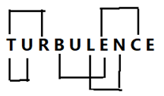S3. Ans. (e)
Sol. Given number – 564854538
After operation – 553753537
Ascending order – 333555577
So, required sum = 5 +5 = 10

S4. Ans. (e)
Sol. Consonants are replaced by its previous letter and vowels to its next letter i.e. BBSVBSF. So, there is no vowel in rearranged word.

S5. Ans. (b)
Sol.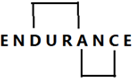S6. Ans. (c)
Sol.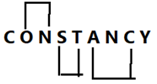S7. Ans. (c)
Sol. Given number – 746587835
After operation – 846688846
Descending order – 888866644
Required difference = 6 – 6 = 0

S8. Ans. (b)
Sol. If we replaced odd positioned letters with their just succeeding letter, then we would get the respective word “OAUIPNBL”. So, the third letter from right end is N.

S9. Ans. (a)
Sol. 1st, 3rd, 5th and 6th letters of word – S, B, P, E
Hence, no meaningful word can be formed.

S10. Ans. (e)
Sol. 2nd, 5th, 6th and 7th letters of word – P, O, R, E
So, three words can be formed – PORE, ROPE, REPO. Hence, X is the correct answer.

S11. Ans. (d)
Sol. Given number – 86475432
After operation – 86497452
Descending order – 98765442
Required sum = 5 + 4 = 9.

S12. Ans.(d):
Sol.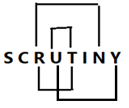S13. Ans. (c)
Sol.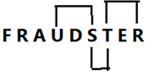S14. Ans. (b)
Sol. 2nd, 5th, 6th and 11th letter of the word – E, R, A and D
Meaningful word is – READ, DEAR and DARE

S15. Ans. (a)
Sol. 1st, 2nd, 4th and 7th letters of word – C, O, C, L
Hence, no meaningful word can be formed.#### Congratulations!Incorrect details? Fill the form again here

•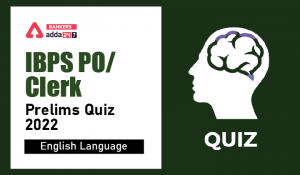English Quizzes For IBPS Clerk/PO Prelim...
•English Quizzes For IBPS Clerk/PO Prelim...
•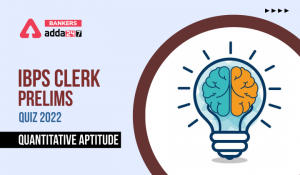Quantitative Aptitude Quiz For IBPS Cler...
•Quantitative Aptitude Quiz For IBPS Cler...
•Reasoning Ability Quiz For IBPS Clerk/PO...
•Quantitative Aptitude Quiz For IBPS Cler...